# 3.4 Basic Properties of the Diamond Structure

The structure depicted in Figure 3.4 consists of two basis atoms and may be thought of as two inter-penetrating face centered cubic (fcc) lattices, one displaced from the other by a translation of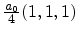along a body diagonal.

 [a][b]In cubic semiconductors such as Si or Ge the two atoms of the basis are identical and the structure is called the diamond structure. If the two basis atoms are different, the structure is called the zinc-blende structure. Many III-V semiconductors such as GaAs, AlsAs, InAs, or InP are of zinc-blende type.

In Figure 3.4 the diamond structure is depicted. The primitive basis vectors and the two atoms atandare highlighted in Figure 3.4b. The basis vectors of the direct Bravais lattice are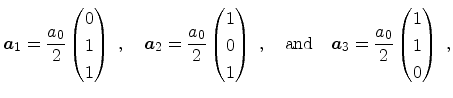(3.21)

wheredenotes the lattice constant of the relaxed lattice. The lattice is invariant under translations involving lattice vectors of the form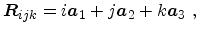(3.22)

where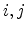and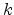are integers. In the unstrained lattice the distance from the basis atom atto its four nearest neighbors is given by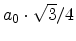.

The basis vectors of the reciprocal lattice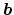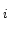are obtained from the relationas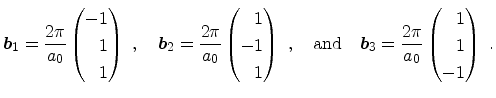(3.23)

General reciprocal lattice vectors are of the form(3.24)

whereand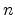are integers.

The first Brillouin zone (BZ) represents the central (Wigner-Seitz) cell of the reciprocal lattice. It contains all points nearest to the enclosed reciprocal lattice point. The boundaries of the first BZ are determined by planes which are perpendicular to the reciprocal lattice vectors pointing from the center of the cell to the 14 lattice points nearest to the origin of the cell at their midpoints. The 14 faces are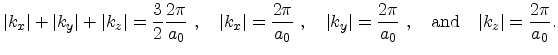(3.25)

 [a]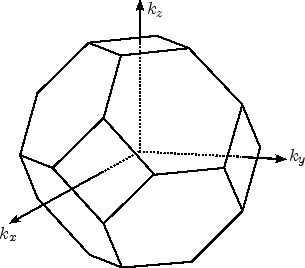[b]In Figure 3.5a the first BZ is depicted. It has a volume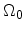of[Singh93].

Due to the translational invariance of the lattice the wave functions and the energy bands are periodic in the reciprocal space and it is sufficient to consider only the first BZ for band structure calculations [Yu03].

The diamond structure is invariant not only under translations, but also under several other symmetry operations such as reflections, rotations, or inversion. These symmetry operations are usually denoted as point operations, since they leave at least one point of the lattice invariant, which is not the case for translations. The set of all point operations for a particular crystal structure forms a group which is denoted as point group. The point group of the diamond structure has 48 symmetry elements which are reflected in the symmetry of the first BZ. A quick examination (see Figure 3.5) shows that the BZ is invariant under various rotations, for example 90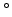rotations about the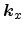,, and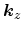axes and under reflections through certain planes. A detailed examination of all 48 point symmetries of the unstrained diamond structure will be given in Section 3.5.2.

The point symmetries of the crystal structure are mirrored in the crystal potential, and hence in the one-particle Hamiltonian used for band structure calculations. Two important consequences for the electron band structure arise:

• Wave functions can be expressed in such a form that they have definite transformation properties under symmetry operations of the crystal. Certain matrix elements of operators can be shown to vanish and selection rules can be deduced, when classifying the wave functions according to their symmetry.
• The energy bands possess the full point symmetry of the point group of the crystal [Yu03,Nowotny98]. The symmetries can be exploited to restrict the band structure calculation to a fraction of onlyth of the first BZ. This fraction is called irreducible wedge of the BZ.

The irreducible wedge of the fcc lattice is depicted in Figure 3.5b. It has six corners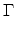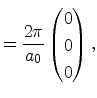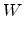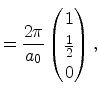(3.26)

connected via symmetry lines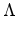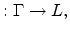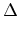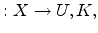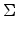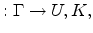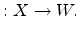(3.27)

By convention upper case Greek letters are used for points and lines inside the first BZ, whereas roman letters denote points and lines on the surface of the first BZ. Here, the points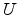andcan be interchanged, since they are equivalent in the reciprocal space (see Section 3.5).
Subsections
E. Ungersboeck: Advanced Modelling Aspects of Modern Strained CMOS Technology